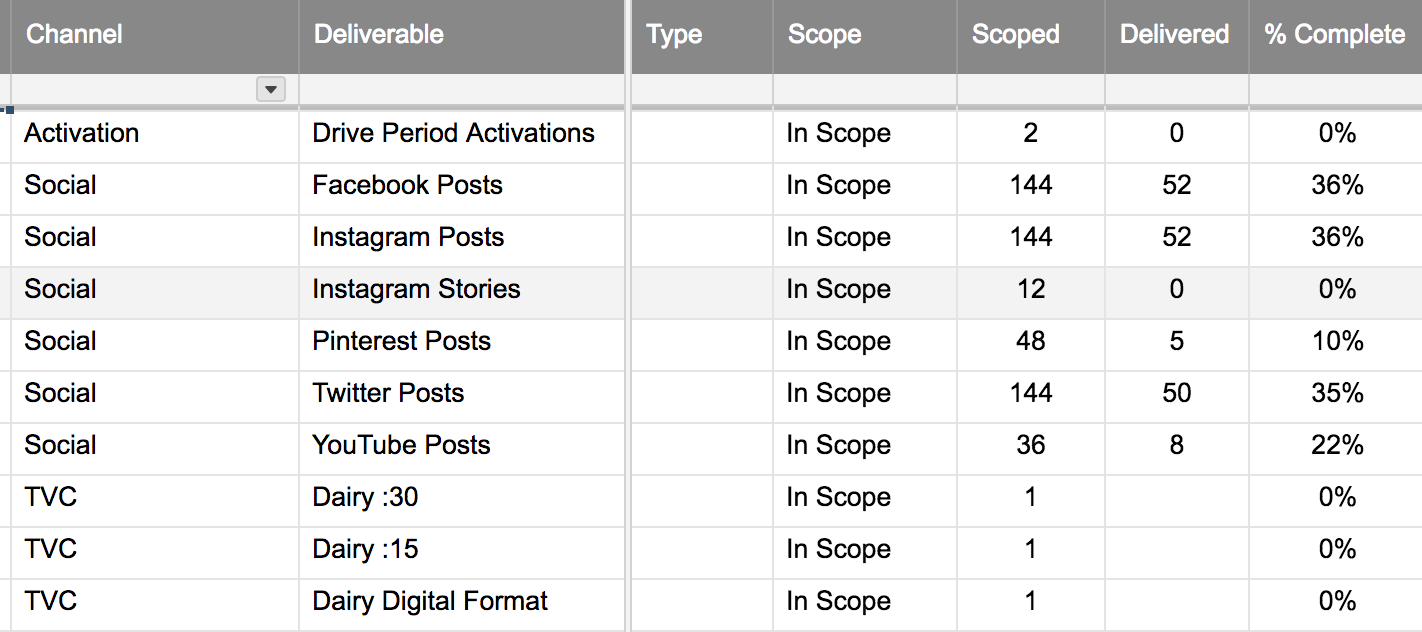# countif help

Hi all,

I'm trying to figure out a way to sum the number of one column if it has the value of another column. The formula I was trying is:

=COUNTIF(Scoped:Scoped, Channel22)

I want to know how many are scoped in the scoped column if the channel header is in row 22.

Any other ideas?

This is unclear to me:

I want to know how many are scoped in the scoped column if the channel header is in row 22.

Your current formula is written for a single cell. It should return the number of times whatever is the value of Channel column, row 22 is found in the cell in the Scoped column.

For example if [Channel]22 was "Not Scoped", then it would count ever "Not Scoped" in the [Scoped] column.

But you wouldn't be asking if it worked.

Craig

• Hi Craig,

If you look at the screenshot attached, I'm trying to total the number of items in the scoped column (as well as delivered and % complete) by channel.

So how many activations are scoped? How many social are delivered? What percent of TVCs have been completed ? Does that make more sense?• =SUMIFS([Scoped]:[Scoped],[Channel]:[Channel],"Activation")

https://help.smartsheet.com/function/sumifs

(Don't use SUMIF, it is a waste of time, eventually)

first argument is what you are totaling (use COUNTIFS for counting)

[Scoped]:[Scoped] is the whole column.

the next arguments come in pairs -- range (which has to be the same size as the range you are summing) and the criteria.

You should be able to swap out the arguments to get the total social delivered.

For the last one, you'll want to sum the # delivered and divide by the # scoped (I suspect). That will be a number 0-1 and then you can format it to percentage.

I hope that helps.

Craig

• Thanks Craig.

It works when I use that formula for ONE channel line (e.g. activation), however when I try to replicate it using another channel, I get "blocked" and "circular."  Do you know what I'm doing wrong?

• That sounds like a location problem. The formula can not be in any of the columns you reference in your formula.

Craig

## Help Article Resources

Want to practice working with formulas directly in Smartsheet?

Check out the Formula Handbook template!